# The TTEST Procedure

#### One-Sample Design

Define the following notation: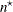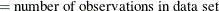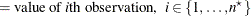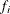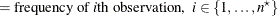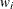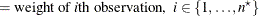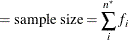##### Normal Data (DIST=NORMAL)

The mean estimate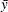, standard deviation estimate s, and standard error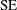are computed as follows: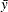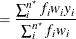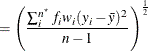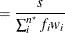The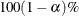confidence interval for the meanis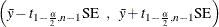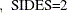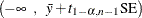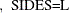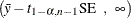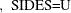The t value for the test is computed as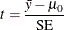The p-value of the test is computed as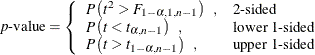The equal-tailed confidence interval for the standard deviation (CI=EQUAL) is based on the acceptance region of the test of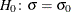that places an equal amount of area (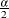) in each tail of the chi-square distribution: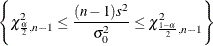The acceptance region can be algebraically manipulated to give the followingconfidence interval for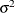: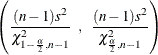Taking the square root of each side yields theCI=EQUAL confidence interval for: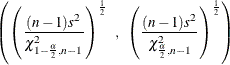The other confidence interval for the standard deviation (CI=UMPU) is derived from the uniformly most powerful unbiased test of(Lehmann, 1986). This test has acceptance region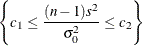where the critical values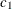and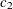satisfy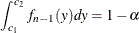and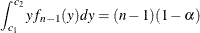where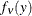is the PDF of the chi-square distribution with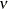degrees of freedom. This acceptance region can be algebraically manipulated to arrive at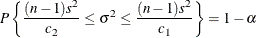whereandsolve the preceding two integrals. To find the area in each tail of the chi-square distribution to which these two critical values correspond, solve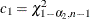and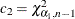for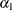and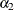; the resultingandsum to. Hence, aconfidence interval foris given by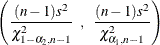Taking the square root of each side yields theCI=UMPU confidence interval for: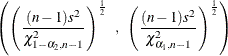##### Lognormal Data (DIST=LOGNORMAL)

The DIST=LOGNORMAL analysis is handled by log-transforming the data and null value, performing a DIST=NORMAL analysis, and then transforming the results back to the original scale. This simple technique is based on the properties of the lognormal distribution as discussed in Johnson, Kotz, and Balakrishnan (1994, Chapter 14).

Taking the natural logarithms of the observation values and the null value, define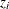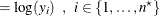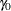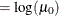First a DIST=NORMAL analysis is performed onin place of. The geometric mean estimate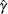and CV estimate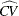of the original lognormal data are computed as follows: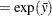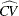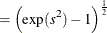The t value and p-value remain the same. The confidence limits for the geometric mean and CV on the original lognormal scale are computed from the confidence limits for the arithmetic mean and standard deviation in the DIST=NORMAL analysis on the log-transformed data, in the same way thatis derived fromandis derived from s.